# types of functions

## What is Function

• A function is a "well-behaved" relation
• A function $f$ is a relation from a non-empty set $A$ to a non-empty set $B$ such that the domain of $f$ is $A$ and no two distinct ordered pairs in $f$ have the same first element.
• For a relation to be a function, there must be only and exactly one $y$ that corresponds to a given $x$
• If $f$ is a function from $A$ to $B$ and $\left( {a,{\rm{ }}b} \right) \in f$, then $f\left( a \right) = b$, where $b$ is called the image of $a$ under $f$ and $a$ is called the preimage of $b$ under$f$.

## Algebra of Real Function

Real Value Function: A function which has all real number or subset of the real number as it domain
Real Valued Function: A function which has all real number or subset of the real number as it range
For functions $f:{\rm{ }}X - > {\bf{R}}$ and $g:{\rm{ }}X - > {\bf{R}}$, we have
$\left( {f + g} \right)\left( x \right) = f\left( x \right) + g\left( x \right),x \in X$
2. Substraction
$\left( {f - g} \right)\left( x \right) = f\left( x \right)-g\left( x \right),x \in X$
3. Multiplication
$\left( {f.g} \right)\left( x \right) = f\left( x \right).g\left( x \right),x \in X$
4. Multiplication by real number $\left( {kf} \right)\left( x \right) = k{\rm{ }}f\left( x \right),x \in X$, where $k$ is a real number.
5. Division
$\frac{f}{g}\left( x \right) = \frac{{f(x)}}{{g(x)}}$
$x \in X$ and $g\left( x \right) \ne 0$

## Type Of Function

We will check here One to One Function or Injective function , onto function or surjective function and Bijective functions

### One to One Function or Injective Function

Let f: A -> B, a function from a set A to a set B, f is called a one-to-one function or injection, if, and only if, for all elements a1 and a2 in A,
if f(a1) = f(a2), then a1 = a2

Equivalently,

if a1 ≠ a2, then f(a1) ≠ f(a2).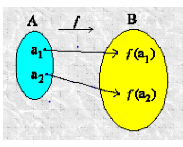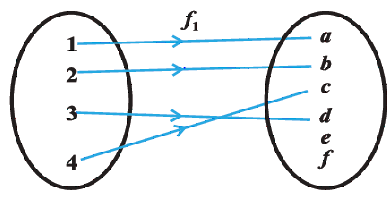A function is not one on one if this condition is met, then it is called many one function
f(a1) = f(a2), then a1 ≠ a2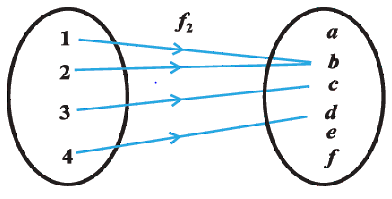#### How to Prove a Function for One to One

(1) We have to prove that
if f(a1) = f(a2), then a1 = a2

(2) Let us take example as
f : R-> R
f(x) =3x-2 for x ∈ R
Then f(a1) =3a1-2
f(a2)=3a2-2

Now f(a1) = f(a2),

3a1-2=3a2-2
or a1 = a2
So our first point is proved,so this is one to one function

#### How to prove a function for Many one 's ( Not one to one)

(1) We have to prove that
if f(a1) = f(a2), then a1≠ a2
(2) We can prove this by giving counter example
f : R-> R
f(x) =x2 for x ∈ R
Now for x=2 f(2)=4
for x=-2 ,f(-2)=4
Now f(2)=f(-2) Hence Many one

### Onto (Surjective ) Function:

A function f: A-> B is said to be onto(surjective) if every element of B is the image of some element of A under f, i,e
for every y ∈ B, there exists a element x in A where f(x)=y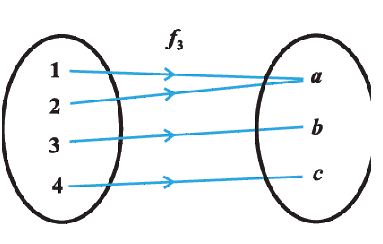#### How to Prove a Function for Onto (Surjectivity):

(1) for every y ∈ B, there exists a element x in A where f(x)=y
(2) Let us take example as
f : R-> R
f(x) =3x-2 for x ∈ R
(3) Let y ∈ R, then we first need to prove that we can find x ∈ R such that f(x)=y
If such real number x exists then y=3x-2
or x=(y+2)/3
Now sum and division of real number is a real number,So x ∈ R
it follows
f((y+2)/3 )= 3(y+2)/3 -2=y

hence f is onto function

(4) if we have to prove that function is not onto,then easiast way would be to take counter examples

### Bijective (One on One and onto) Function

A function is said to be Bijective if it is both one on one and onto function.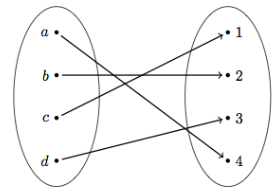#### How to Prove a Function for Bijectivity

To prove a function is bijective, you need to prove that it is injective and also surjective.
"Injective" means no two elements in the domain of the function gets mapped to the same image.The method has been already described above.
"Surjective" means that any element in the range of the function is hit by the function.The method has been already described above.

## Solved examples

Question 1
A function f : N -> N, given by f(x) = 2x, Show that it is one-one but not onto
Solution :
One-one Check
for f(x1) = f(x2)
Or x1= 2x1
or x1= x2
So it is one-one
Onto Check
f is not onto, as for 1 ∈ N, there does not exist any x in N such that f(x) =2 x = 1

Question 2
A function f : R -> R, given by f(x) = x2, Find out if it is one-one and onto
Solution :
One-one Check f(-1) = 1
f(1) =1
So it is not one-one
Onto Check
f is not onto, as for -1 ∈ R, there does not exist any x in R such that f(x) = x2 = -1

Question 3
Show that an onto function f : {1, 2, 3} -> {1, 2, 3} is always one-one.
Solution :
Lets take this by contradiction method
So we assume that f is not one-one.
Then there exists two elements, say 1 and 2 in the domain whose image in the co-domain is same. Also, the image of 3 under f can be only one element. Therefore, the range set can have at the most two elements of the co-domain {1, 2, 3}, showing that f is not onto, a contradiction. Hence, f must be one-one.
Question 3
Show that a one-one function f : {1, 2, 3} → {1, 2, 3} must be onto.
Solution :
Since f is one-one, three elements of {1, 2, 3} must be taken to 3 different elements of the co-domain {1, 2, 3} under f. Hence, f has to be onto

Go back to Class 12 Main Page using below links